Courses

# Programming in C++, C & Java - 2

## 15 Questions MCQ Test GATE Computer Science Engineering(CSE) 2022 Mock Test Series | Programming in C++, C & Java - 2

Description
This mock test of Programming in C++, C & Java - 2 for Computer Science Engineering (CSE) helps you for every Computer Science Engineering (CSE) entrance exam. This contains 15 Multiple Choice Questions for Computer Science Engineering (CSE) Programming in C++, C & Java - 2 (mcq) to study with solutions a complete question bank. The solved questions answers in this Programming in C++, C & Java - 2 quiz give you a good mix of easy questions and tough questions. Computer Science Engineering (CSE) students definitely take this Programming in C++, C & Java - 2 exercise for a better result in the exam. You can find other Programming in C++, C & Java - 2 extra questions, long questions & short questions for Computer Science Engineering (CSE) on EduRev as well by searching above.
QUESTION: 1

### What does the following C-statement declare?  int (*f) (int *);

Solution:

int (*f) (int*);
return type int, (*f) is a pointer to a function the argument is (int*) an integer pointer So, int (*f) (int*) means a pointer to a function that takes an integer pointer as an argument and returns an integer.

QUESTION: 2

### An Abstract Data Type (ADT) is

Solution:

The abstract data type (ADT) refers to a programmer defined data type together with a set of operations that can be performed on that data so the choice (c) is correct.

QUESTION: 3

### A common property of logic programming languages and functional languages is

Solution:

A common property of logic programming languages and functional languages is both are declarative because we declare any statement before we will use it.

QUESTION: 4

Which of the following are essential features of an object-oriented programming languages?
1. Abstraction and encapsulation
2. Strictiy-typedness
3. Type-safe property coupled with sub-type rule
4. Polymorphism in the presence of inheritance

Solution:

Object oriented programming language is Object based PL + Abstraction + Inheritance.
The last two (abstraction and inheritance) are must for any L to be OOPL.

QUESTION: 5

Early binding refers to a binding performed at compile time and late binding refers to a binding performed at execution time. Consider the following statements:
(i) Static scope facilitates w1 bindings.
(ii) Dynamic scope requires w2 bindings.
(iii) Early bindings w3 execution efficiency.
(iv) Late bindings w4 execution efficiency.
The right choices of w1, w2, w3 and w4 (in that order) are

Solution:

(i) Static scoping facilitate early binding because it is done at compile time.
(ii) Dynamic scoping require late binding because it is done at runt time.
(iii) Early binding increases execution efficiency because both necessary and uncessary things are load at compile time.
(iv) Late binding decrease execution efficiency because only necessary things are loaded when they are needed.

QUESTION: 6

Match the programming paradigms and languages given in the following table: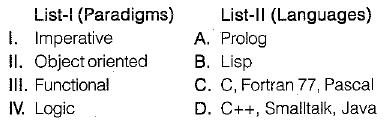Codes:

Solution:

Imperative: C, Fortran 77, Pascal
Object oriented : C + + , Smalltalk, Java
Functional: Lisp
Logic: Prolog

QUESTION: 7

Which combination of the integer variables x, y and z makes the variable a get the value 4 in the following expression?
a = (x > y) ? ((x > z) ? x : z) : ((y > z) ? y : z)

Solution:

a = (x < y) ? ((x < z) ? x : z) : ((y > z) ? y: z)
In C “ ? is a ternary operator the syntax is (exp1 ? exp2 : exp3). it means if exp1 is true print exp2 else exp3.
Let x = 3, y = 4, z = 2.
⇒ a = (3 > 4) ? ((3 > 2) ? 3 : 2 ) : ((4 > 2) ? 4 : 2)
a = (3 > 4) ? 3 : 4
a = 4

QUESTION: 8

What does the following program print?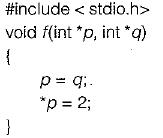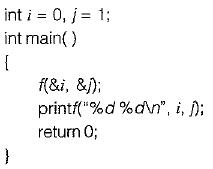Solution:

In the given program it begin from main function, i and j are globally initialized by 0 and 1. So when we call function f(& i, & j) the address of i and j are passed.
when p = q and *p = 2 means
*q = 2, so value of *q is passed.
and value of *q return to j.
Value of i and j are 0 and 2 respected.
So print i (“ % d % d ”, i, j) gives output (0, 2).

QUESTION: 9

What is the value printed by the following C program?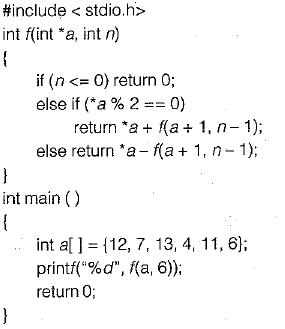Solution: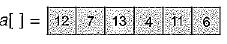So f(a, 6) → first this call
Thus is the number stored in the array and we can access these by using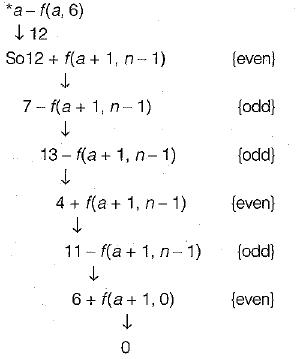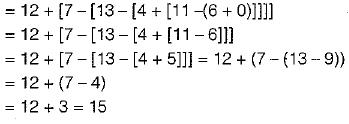QUESTION: 10

What does the following fragment of C program print?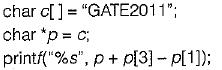Solution: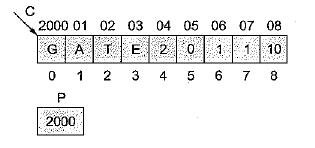P + P - P = 2000 + 69 - 65
= 2004
⇒ 2011 string will be printed.
(From 2004 Address)

QUESTION: 11

Consider the following program in C language: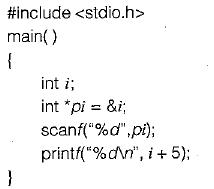Which one of the following statements is TRUE?

Solution: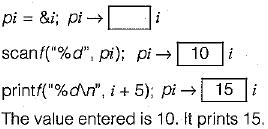QUESTION: 12

Consider the C function given below: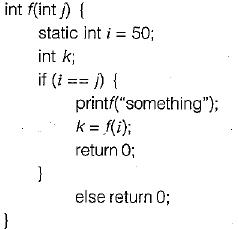Which one of the following is TRUE?

Solution:

f(50) goes into infinite loop by calling f(i) recursively for i = j = 50.

QUESTION: 13

Consider the following C program segment: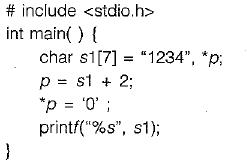What will be printed by the program?

Solution: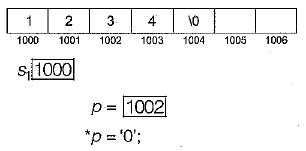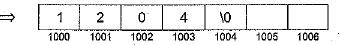Therefore the output is 1204

QUESTION: 14

Which of the following comments are not true?
1. C provides no input-output features
2. C provides no file access features
3. C borrowed most of its ideas from BCPL
4. C provides no features to manipulate composite objects

Solution:

BCPL: Basic Combined Programming Language primary used for system software and called simply B.

QUESTION: 15

Which of the following are not keywords in C?

Solution:

"if" is keyword in C not “IF”.